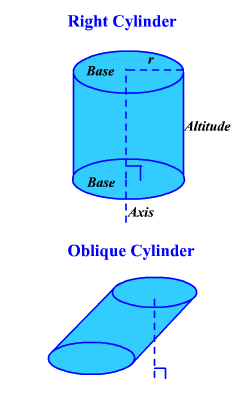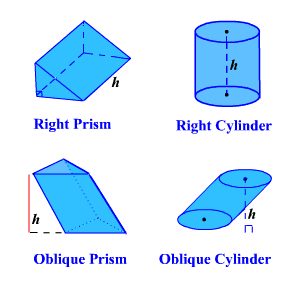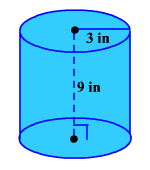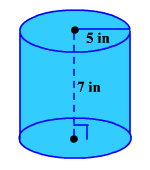# Cylinder

A cylinder is a solid composed of two congruent circles in parallel planes, their interiors and all the line segments parallel to the segment containing the centers of both circles with endpoints on the circular regions.

The circles and their interiors are the bases .  The radius of the cylinder is the radius of a base.  The altitude of the cylinder is a perpendicular segment from the plane of one base to the plane of the other and the height of the cylinder is the length of the altitude.

The axis of a cylinder is the segment containing the centers of the two bases.  If the axis is perpendicular to the planes of the two bases, the cylinder is a right cylinder ; otherwise, it is an oblique cylinder.A cylinder is closely related to a prism , so the formulas for their surface areas are related.Remember the formulas for the lateral surface area of a prism isand the total surface area is.  Since the base of a cylinder is a circle, we substituteforandforwhereis the radius of the base of the cylinder.

So, the formula for the lateral surface area of a cylinder is. (The lateral surface of a cylinder when unwrapped and laid flat is a rectangle whose dimensions are the height of the cylinder and the circumference of the base.)

Example 1:

Find the lateral surface area of a cylinder with a base radius ofinches and a height ofinches.The general formula for the total surface area of a cylinder is.

Example 2:

Find the total surface area of a cylinder with a base radius ofinches and a height ofinches.The volume of a cylinder equals the area of a base times the height of the cylinder
().

Example 3:

Find the volume of a cylinder with radiuscm and heightcm.